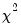edftest
Computes goodness-of-fit tests based on the empirical distribution function.
Syntax
series_name.edftest(options)
Options
General Options

 dist=arg (default=”nomal”) Distribution to test: “normal” (Normal distribution), “chisq” (Chi-square distribution), “exp” (Exponential distribution), “xmax” (Extreme Value - Type I maximum), “xmin” (Extreme Value Type I minimum), “gamma” (Gamma), “logit” (Logistic), “pareto” (Pareto), “uniform” (Uniform). p1=number Specify the value of the first parameter of the distribution (as it appears in the dialog). If this option is not specified, the first parameter will be estimated. p2=number Specify the value of the second parameter of the distribution (as it appears in the dialog). If this option is not specified, the second parameter will be estimated. p3=number Specify the value of the third parameter of the distribution (as it appears in the dialog). If this option is not specified, the third parameter will be estimated. prompt Force the dialog to appear from within a program. p Print test results.
Estimation Options
The following options apply if iterative estimation of parameters is required:

 b Use Berndt-Hall-Hall-Hausman (BHHH) algorithm. The default is Marquardt. m=integer Maximum number of iterations. c=number Set convergence criterion. The criterion is based upon the maximum of the percentage changes in the scaled coefficients. showopts / ‑showopts [Do / do not] display the starting coefficient values and estimation options in the estimation output. s Take starting values from the C coefficient vector. By default, EViews uses distribution specific starting values that typically are based on the method of the moments.
Examples
x.edftest
uses the default settings to test whether the series x comes from a normal distribution. Both the location and scale parameters are estimated from the data in X.
freeze(tab1) x.edftest(type=chisq, p1=5)
tests whether the series x comes from adistribution with 5 degrees of freedom. The output is stored as a table object TAB1.
Cross-references
See “Empirical Distribution Tests” for a description of the goodness-of-fit tests.